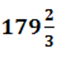Newbie

# If the volume of a sphere is 179 2/3 cube cm, Find its radius and surface area.

• 1

ICSE Board Question Based on Mensuration Chapter of M.L Aggarwal for class10
In this question If the volume of a sphere is given, Find its radius and surface area.
This is the Question Number 13, Exercise 17.3 of M.L Aggarwal.

Share

1.This answer was edited.

Given volume of the sphere iscube cm

(4/3)πr= 539/3

(4/3)×(22/7)×r= 539/3

r3 = (539×3×7)/(4×22×3)

r3 = 49×7/8

r3 = 7×7×7/(2×2×2)

Taking cube root on both sides, we get

r = 7/2 = 3.5 cm

Surface area of the sphere = 4πr2

= (4/3)×(22/7)×(7/2)2

= 22×7

= 154 cm2

Hence the radius and the surface area of the sphere is 3.5 cm and 154 cm2 respectively.

• 3